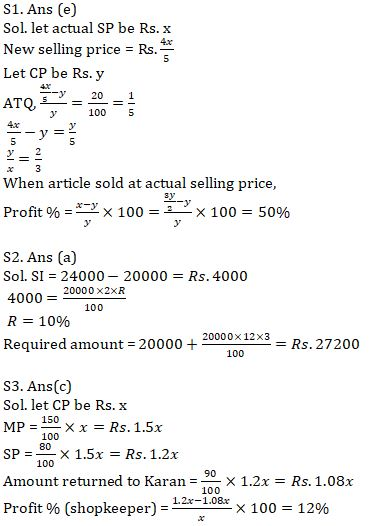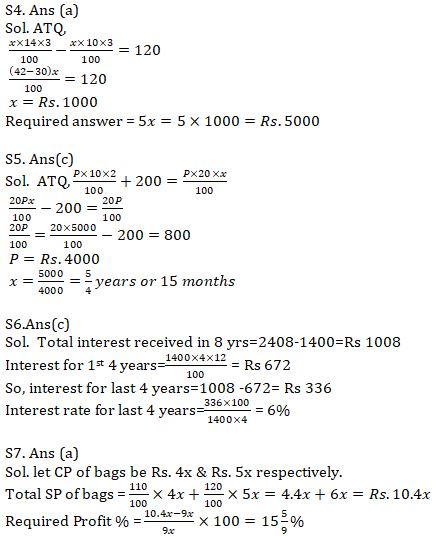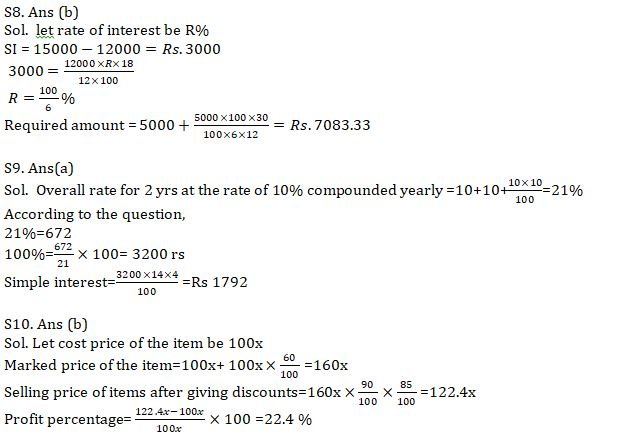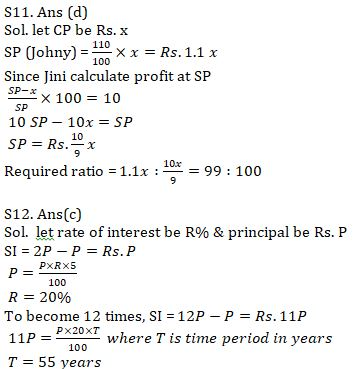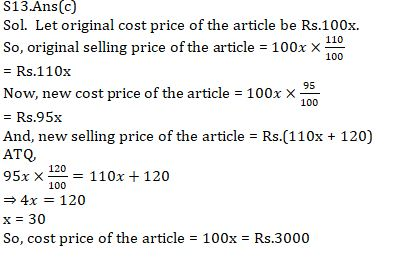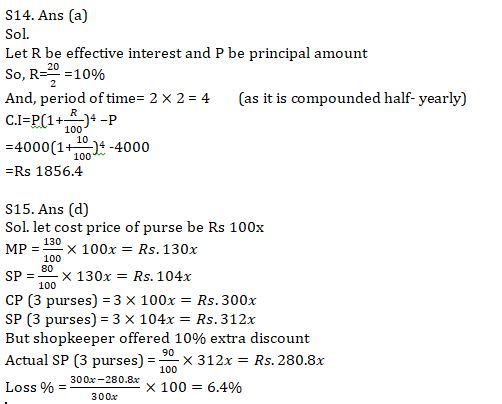Latest Banking jobs   »   Quantitative Aptitude Quiz For IBPS Clerk...

# Quantitative Aptitude Quiz For IBPS Clerk Prelims 2021- 3rd August

Q1. An article when sold at 4/5 of its original selling price, gives a profit of 20%. Find the profit % when the same article is sold at its actual selling price.
(a) 15 %
(b) 20 %
(c) 25%
(d) 22 %
(e) None of these

Q2. An amount of Rs. 20000 when invested at R% simple interest for 2 years becomes Rs. 24000. What will it become in 3 years if invested at (R+2)%? (in Rs.)
(a) 27200
(b) 26300
(c) 25200
(d) 27400
(e) 28100

Q3. Karan purchased an article marked up by 50% at a discount of 20% but later he found that the article was having a defect so he decided to return it but the shopkeeper returned him only 90% of what he had paid. What is profit/loss (in %)of the shopkeeper in the whole transaction?
(a) 8
(b) 10
(c) 12
(d) 15
(e) None of these

Q4. A sum of Rs. x was invested at 10% simple interest for 3 years. If the same sum was invested at 4% more for same period, then it would have fetched Rs. 120 more. Find the value of 5x. (in Rs.)
(a) 5000
(b) 4800
(c) 3600
(d) 5500
(e) 4000

Q5. A sum of Rs. P was invested at 10% for 2 years at simple interest. If the same sum was invested at 20% for ‘x’ years, it would have fetched Rs. 200 more. Find ‘x’ if Px = 5000. (value of x is given in months)
(a) 12
(b) 18
(c) 15
(d) Cannot be determined
(e) None of these

Q6. A sum of Rs 1400 becomes Rs 2408 in 8 yrs at simple interest, then find the rate of interest for last 4 yrs,if the interest rate for 1st 4 yrs is 12% per annum ?
(a) 8 %
(b) 10 %
(c) 6%
(d) 4 %
(e) None of these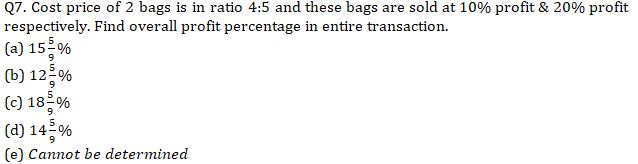Q8. Rs. 12000 becomes Rs. 15000 in 18 months at a certain rate of interest at simple interest. Find amount if Rs. 5000 invested at same rate for 30 months at simple interest.
(a) Rs. 7883.33
(b) Rs. 7083.33
(c) Rs. 7279.80
(d) Rs. 7173.33
(e) None of these

Q9.The interest earned on an amount after 2 yrs at 10 % per annum compounded yearly is Rs 672. Find the interest earned on same amount after 4 yr at 14 % per annum at simple interest ?
(a) Rs 1792
(b) Rs 1864
(c) Rs 1912
(d) Rs 1754
(e) Rs 1720

Q10.If the shopkeeper marked the price of an item 60% above the cost price and then gives two successive discount of 10% and 15% respectively, then find the profit percentage of the shopkeeper on selling the item?
(a) 25.4%
(b) 22.4%
(c) 20%
(d) 28.5%
(e) 32%

Q11. Johny calculates his profit at cost price while Jini at selling price. If cost price is same for all and everyone calculate their profit as 10%. Find ratio of selling price.
(a) 100:111
(b) 10:11
(c) 10:101
(d) 99:100
(e) Cannot be determined

Q12. An amount doubles in 5 years at simple interest. In what time will it become 12 times of itself at same rate? (in years)
(a) 30
(b) 50
(c) 55
(d) 36
(e) None of these

Q13.Aakash sells an article at a profit of 10%.Had he bought it for 5% less and sold it for 120 rs more then he would have gained 20% profit. What is the cost price of the article ?
(a)Rs 2500
(b) Rs 4000
(c) Rs 3000
(d) Rs 3500
(e) Rs 2000

Q14. An amount of 4000 rs is invested at 20% per annum for 2 yrs at compound interest compounding half-yearly, then find the total interest amount received after 2 yrs ?
(a) Rs 1856.4
(b) Rs 1812.4
(c) Rs 1882.4
(d) Rs 1912.4
(e) None of these

Q15. Anu bought a purse at a discount of 20% which was marked at 30% higher than cost price. A customer gets 10% extra discount on purchase of more than two purse. If Anu purchased 3 such purses, find profit or loss percent of shopkeeper.
(a) 4% loss
(b) 4% profit
(c) 6.4% profit
(d) 6.4% loss
(e) None of these

Solutions